# Calculate The Number of Days/Weeks/Months/Years between Two Dates in Excel

There might be a lot of reasons that you need to calculate the number of days between two dates in excel or number of weeks, months and years between two dates in Excel. If you have been given a task which you have to complete within a fixed time, knowing the number of workdays or weekends is a smart thing.

In this article, I will demonstrate different formulas and functions for calculating the number of days, weeks, years, weekends and workdays in excel.

## Subtracting two dates in excel

Subtracting two days in excel is like subtracting between numbers. Two subtract dates you simply need to perform the subtraction between the cells containing dates. The following picture shows how it is done.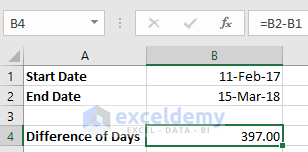Here, the subtraction between 2 dates gives us the result as the difference of days between 2 dates.

Note: While performing this simple calculation you may find error values. To fix the errors, make sure your both dates are in the same date format. Change the format of the cell which gives you the difference of the days between 2 dates as the General format. For performing cell formatting click on the drop-down of the Number format in the Number group of Home tab.

## Performing Excel Today Minus Date Calculation

If you want to subtract the number of days between any earlier-date and present date, use the below formula.

=TODAY ()-Earlier-Date

Again, if you want to subtract the number of days between a later-date and the present date, use the below formula.

=Later-Date-TODAY ()

The following picture shows these two calculations.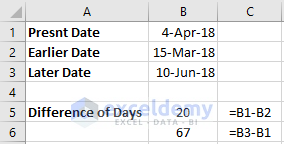Note: TODAY function recalculates the date every day. So, if you open your workbook after some days the TODAY function updates the date automatically.

## Excel Formula to Count Days from Date using DAYS Function

Excel has a built-in function to calculate days from two dates. This function can be used with other functions to set different formulas for calculating the number of days between 2 dates. The following picture states different formulas and formulated result of counting. The main function that is used in every case is the DAYS function. The following picture shows the different process of using the DAYS function to calculate number of days between two dates in excel.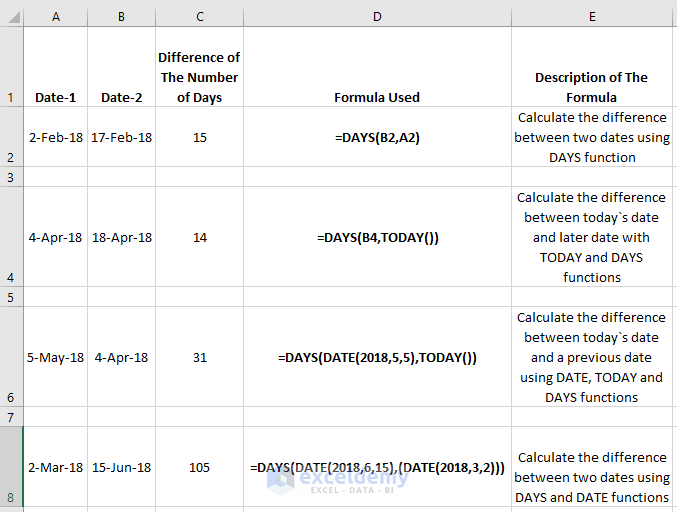Here the formulas which have been used are given below. Copy this formula in your worksheet to perform these calculations. Don`t forget to insert the dates. You can also use the DAYS360 function which is programmed to assume a year as 360 days. So, instead of assuming 365 days of a year it will assume 360 days.

• `=DAYS(B2,A2)`
• `=DAYS(B4,TODAY())`
• `=DAYS(DATE(2018,5,5),TODAY())`
• `=DAYS(DATE(2018,6,15),(DATE(2018,3,2)))`

## Calculate Number of Days Between Two Dates in Excel using DATEDIF Function

Like the DAYS function, DATEDIF function can also calculate the difference between two dates. The difference is, you need to put an extra unit “d” with the function while calculating the number of days. This function is hidden by Microsoft so you cannot find it in the function list or in the formula list. The formula uses the syntax, DATEDIF (start-date, end-date, unit). The following picture shows the different process of using the DATEDIF function to calculate the number of days between two dates in excel.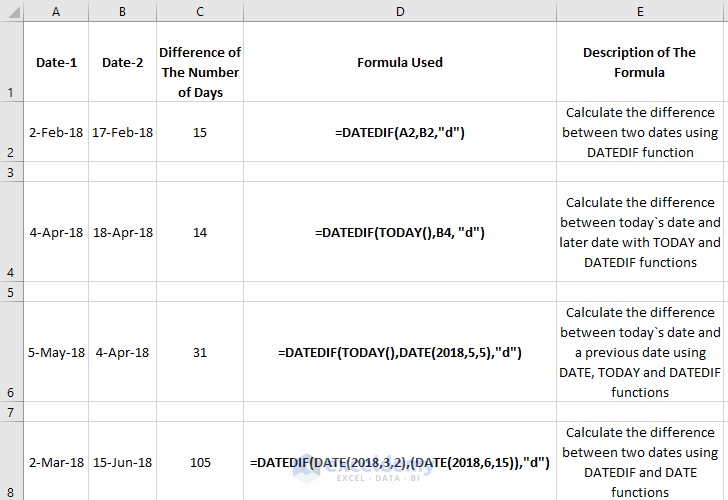Here the functions which have been used are given below.

• ``=DATEDIF(A2,B2,"d")``
• ``=DATEDIF(TODAY(),B4, "d")``
• ``=DATEDIF(TODAY(),DATE(2018,5,5),"d")``
• ``=DATEDIF(DATE(2018,3,2),(DATE(2018,6,15)),"d") ``

Note: As you can see, this example and the previous example uses the same dates. The only difference is in the formulas. In DATEDIF if function the end-date should be greater than the start-date otherwise you will find a value indicating an error value of #NUM!

## Counting Days in Excel Ignoring Months/Years

If your observation has two dates with two different years and in your calculation, you want to ignore months or years, you can do this by using the same DATEDIF function that I used in the previous example. The only difference will be in the unit place.

The below picture shows the number of days between two dates in excel which ignores years and months.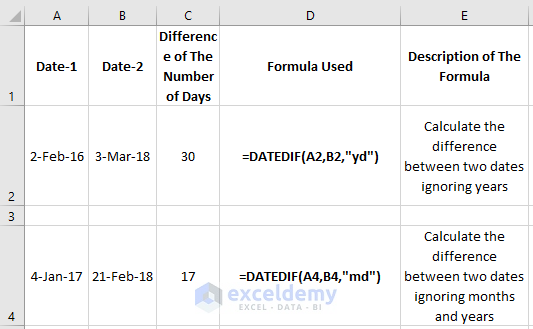`Here the formula `=DATEDIF(A2,B2,"yd")``

ignores the number of years while counting days and

``=DATEDIF(A4,B4,"md")``

ignores both the months and years while counting days.

Similarly, the unit `"ym"` can be used to calculate months while ignoring years and days.

## Counting the Number of Weeks Between Two Dates in Excel

To calculate the number of weeks between 2 dates you need to divide the formula by 7 which calculates the number of days. Just follow the procedure of the below picture to find the number of weeks.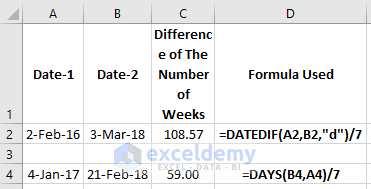Here you can see the decimal numbers as the number is not rounded up.

The formulas that I have used here are,

``=DATEDIF(A2,B2,"d")/7 and =DAYS(B4,A4)/7``

Note: Don’t forget to format the result as number format otherwise it will not show any value.

## Calculate the Number of Months Between Two Dates in Excel

To calculate the number of months you can apply any one of the below formulas. They are very easy calculations but make sure to use them properly as violating the syntax can give you an error value. The following picture shows how the months between 2 dates are calculated.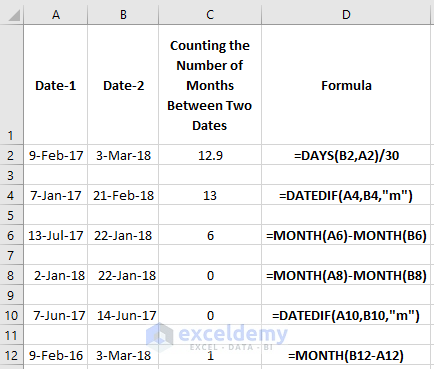The formulas which give the proper result in this example are-

• `=DAYS(B2,A2)/30`
• ``=DATEDIF(A4,B4,"m")``
• `=MONTH(A6)-MONTH(B6)`

In cell C8 and C10 where the MONTH and DATEDIF functions are used, you can see the result is 0 in those two cells. This happens when a full cycle of a month is not complete. Violating the syntax may also give you a negative and error result. In cell C12 you are not seeing an accurate result, because the MONTH function calculates and returns the months within a year. When it is above 12 it starts to calculate the differences from the beginning.

Note: Here the DAYS function will give you a decimal value because it is designed to return the number of days in Excel. Also, the number of days in a month can vary within a year.

Calculating the months between 2 dates becomes complicated when you have a confusion whether to count the months as partial months or full months. This is where the rounding up and rounding down of months is required. The below picture shows how the rounding up and rounding down to months are done.For rounding up I used the formula

`=(YEAR(B2)-YEAR(A2))*12+MONTH(B2)-MONTH(A2)`

and for rounding down I used the formula

`=IF(DAY(B4)>=DAY(A4),0,-1)+(YEAR(B4)-YEAR(A4))*12+MONTH(B4)-MONTH(A4)`

## Calculate the Number of Years Between Two Dates in Excel

Just like the previous example counting the number of years between 2 dates uses the same formulas with some modification. Here I will divide the result by 365 which uses the DAYS function. Instead of MONTH, I will use the YEAR function and in the DATEDIF function where the unit “M” is used. I will use the unit “y” to determine the number of years. The below picture shows you the formulas and calculation of the differences between 2 dates.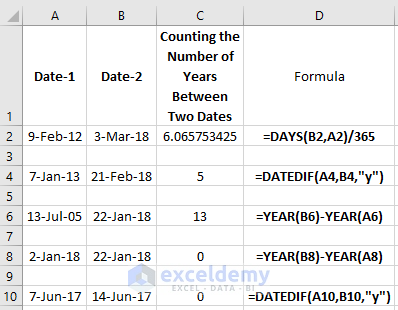The formulas that have been used are given below.

• `=DAYS(B2,A2)/365`
• ``=DATEDIF(A4,B4, "y")``
• `=YEAR(B6)-YEAR(A6)`
• `=YEAR(B8)-YEAR(A8)`
• ``=DATEDIF(A10,B10, "y")``

Just like the previous example, I intentionally produce the result 0 again to tell you that if your calculation doesn’t complete a full cycle of a year you will find a 0 result. Again, the violation of syntax may also give you a negative and error result.

Note: Here the DAYS function again will give you a decimal value because it is designed to return the number of days in Excel. It`s not ideal to use this function while calculating the number of years and months in excel.

## Calculate the Number of Working Days in Excel

You can easily find the number of total working days between 2 dates by using the NETWORKDAYS and NETWORKDAYS.INTL functions. The difference between these two functions is that the NETWORKDAYS is designed to exclude Saturday and Sunday as weekend days by default but in the NETWORKDAYS.INTL functions you can define the weekends.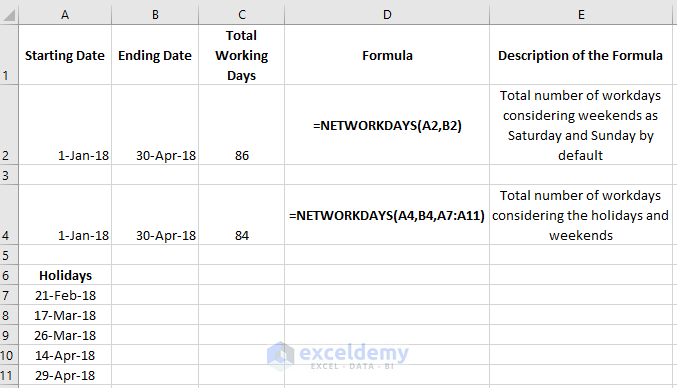In the above picture, you can see the number of workdays between 2 days. The formulas that have been used in this calculation are

`=NETWORKDAYS(A2,B2)`

and

`=NETWORKDAYS(A4,B4,A7:A11)`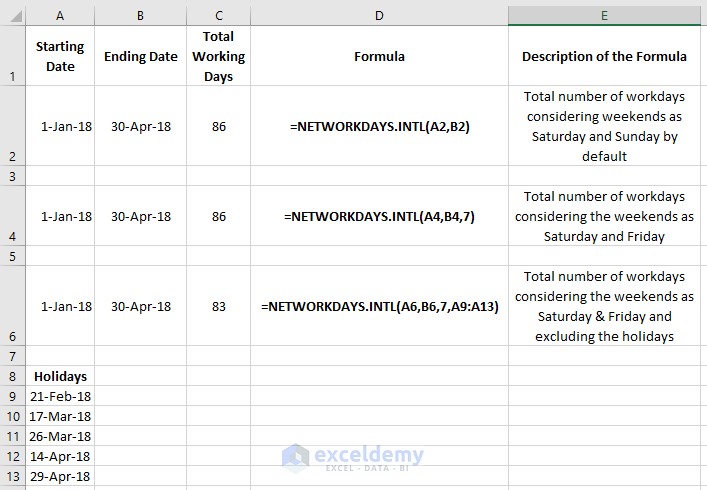In the above table, you can see the number of workdays using the NETWORKDAYS.INTL function. The formulas that have been used in this calculation are given below.

• `=NETWORKDAYS.INTL(A2,B2)`
• `=NETWORKDAYS.INTL(A4,B4,7)`
• `=NETWORKDAYS.INTL(A6,B6,7,A9:A13)`

For defining the weekends in the NETWORKDAYS.INTL formula you can follow the following table.

Weekend daysWeekend number
Saturday & Sunday1 (default)
Sunday & Monday2
Monday & Tuesday3
Tuesday & Wednesday4
Wednesday & Thursday5
Thursday & Friday6
Friday, Saturday7
Sunday only11
Monday only12
Tuesday only13
Wednesday only14
Thursday only15
Friday only16
Saturday only17

## Calculate the Number of Weekends in Excel Between Two Dates

To calculate the number of weekends between 2 dates just insert the formula

`=SUM(INT((WEEKDAY(A2-{3,7})+B2-A2)/7))`

where B2>A2. The following picture shows the result of the number of weekends between 2 dates.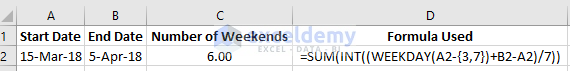## Add/Subtract Days to Date in Excel Excluding Weekends & Holidays

Suppose you want to find the end date of a project that you are working on. You can do this by using the WORKDAY & WORKDAY.INTL functions. In WORKDAY function, Saturday and Sunday are considered as weekends by default. You can manually put the weekends in the WORKDAY.INTL function. In both the functions, the holidays can also be inserted while calculating the end date. The following picture shows the result and formula that has been used to calculate the end date of a project using the WORKDAY function.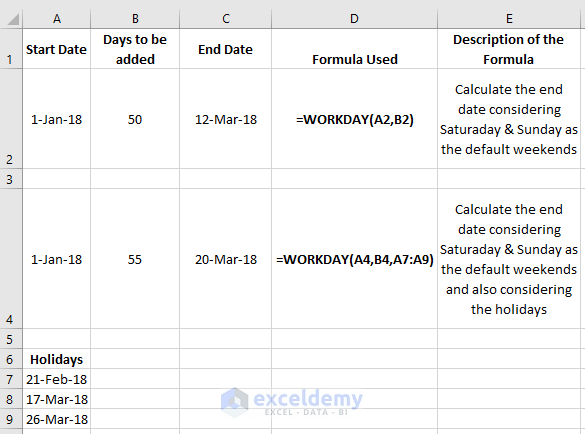In the above picture, the formulas which have been used are

• `=WORKDAY(A2,B2)`
• `=WORKDAY(A4,B4,A7:A9)`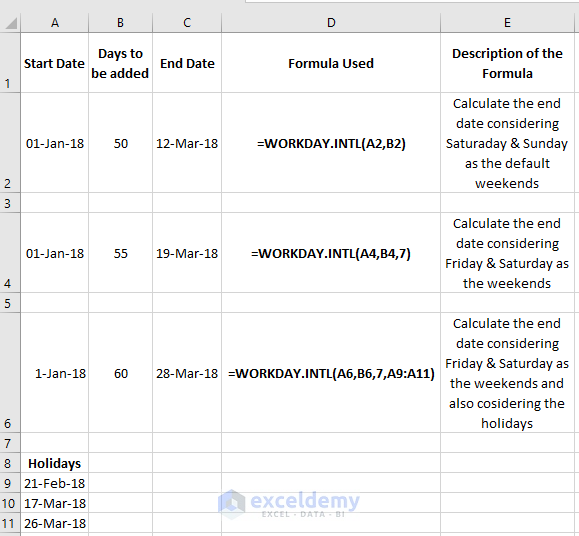The above picture shows the end date calculation using the WORKDAY.INTL function. Here the formulas which have been used are stated as follows.

• `=WORKDAY.INTL(A2,B2)`
• `=WORKDAY.INTL(A4,B4,7)`
• `=WORKDAY.INTL(A6,B6,7,A9:A11)`

You can also subtract days using these two functions to find any previous date. To do this, you need to use a negative number as the days which will subtract the number of days from the date. The following picture shows how it is done.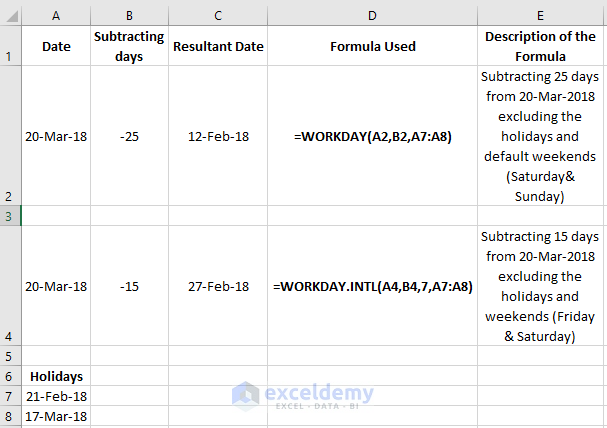Note: Make sure to format the cells properly as you may not find a proper value at first. Format the resultant cell in date format for finding a proper date result.

## Add/Subtract Months to a Date

To add and subtract months to a date you can use the EDATE function. If you want to find the end of the month after adding the months to a date or subtracting the months from a date, you can use the EOMONTH function. The following picture shows how it can be done.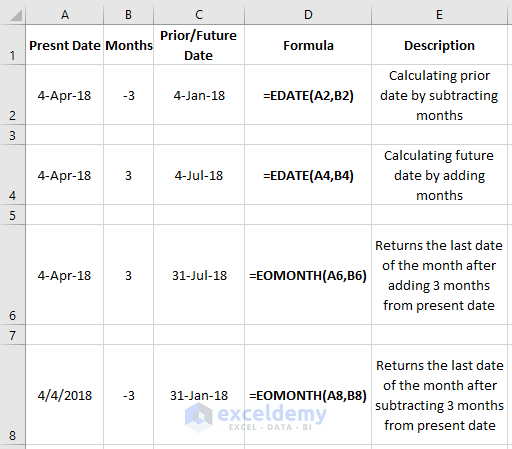The formulas that has been used here are given below.

• `=EDATE(A2,B2)`
• `=EDATE(A4,B4)`
• `=EOMONTH (A6,B6)`
• `=EOMONTH (A8,B8)`

## Add/Subtract Years to a Date in Excel

For adding years to a date and subtracting years from a date to get another date, you can use the 3 functions altogether. The following picture shows how it is done.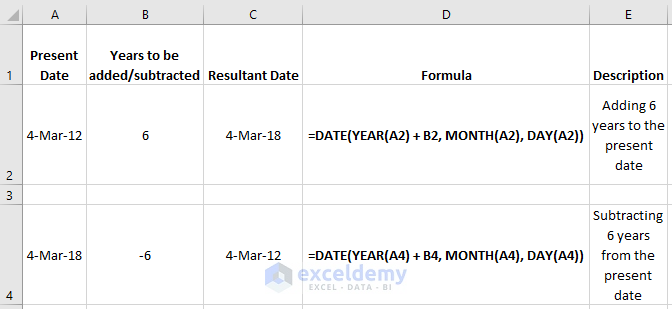The formula for adding or subtracting the years is,

=DATE(YEAR(Cell-reference-of-the-date) + N-years, MONTH(Cell-reference-of-the-date), DAY(Cell-reference-of-the-date))

## How to Calculate The Years of Service in Excel

To calculate the difference between 2 dates which will result in the days, months and years you can use the following formula.

``=DATEDIF(A3,B3,"y")&" years,"&DATEDIF(A3,B3,"ym")&" months,"&DATEDIF(A3,B3,"md")&" days"``

By doing this you can determine the years of service of a person in a company or organization.

The following picture shows the result of the above formula.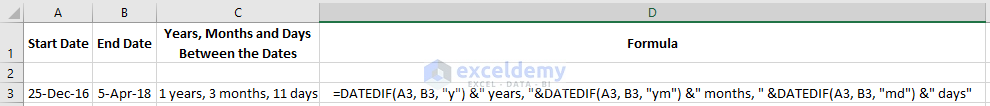## How to Calculate Age in Excel

For calculating a person`s age the same above formula can be used where instead of the end date you should use the present date. The present date can be generated using the TODAY function. In this example, I just calculated my age using the TODAY and DATEDIF function. For calculating the age as years I used the formula

``=DATEDIF(A2, TODAY(), "y")``

If you want to find your age in years, date and months formate just use the below formula.

``=DATEDIF(A4,TODAY(),"y") & " Years, " & DATEDIF(A4,TODAY(),"ym") & " Months, " & DATEDIF(A4,TODAY(),"md") & " Days"``

The below picture shows the age in both the formats that I just discussed.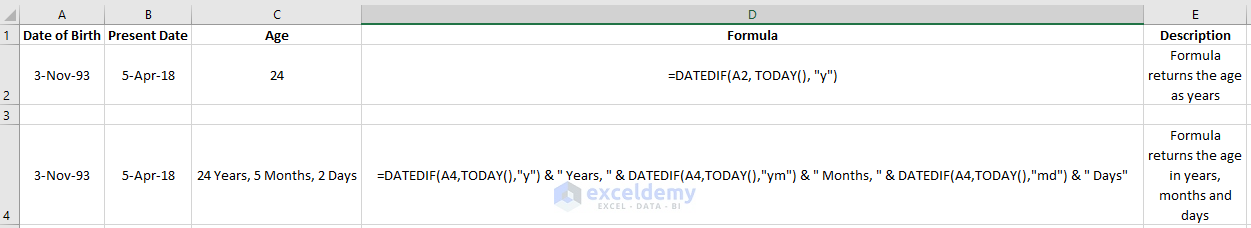Click the picture for a full view

## Conclusion

The main aim of this article was to calculate the days, months, years between two dates. While doing these, I also calculated the age, workdays and other calculations related to the topic. While performing different calculations I came across different date functions. These functions make your calculation smooth and fast.

Click on the link below to download the excel file which includes all the calculation provided in this article.

Calculation of the Number of Days-Weeks-Months and Years Between Two Dates in Excel

I hope this article will be very useful to you. Stay connected for more articles.

#### Siam Hasan Khan

Hello! Welcome to my Profile. Here I will be posting articles related to Microsoft Excel. I have completed my BSc in Electrical and Electronic Engineering from American International University-Bangladesh. I am a diligent, goal-oriented engineer with an immense thirst for knowledge and attitude to grow continuously. Continuous improvement and life-long learning is my motto.